0收藏

@[toc]

# 1 实验题目

π= 4✖️（圆内的点数）/（总点数）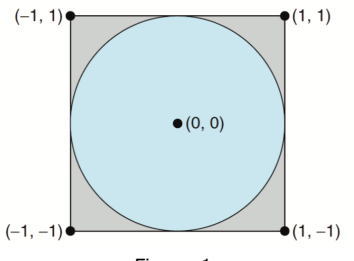（1）请编写一个多线程版的算法，它创建一组子线程，每个子线程产生一组随机点，并确定点是否落在圆内。每个子线程更新所有落在园内的点的全局计数。当所有子线程计算完毕后，使用父线程计算π。（通过采用互斥锁，保护对共享全局变量的更新）？
（2）针对题目（1）请考虑一下，该怎样来提高计算π的性能（速度）？请实现相关的思想或算法的代码，并给出分析说明和实验数据证明！

# 3分析思路

①蒙特卡洛的本质是统计方法，所以必然要获取大量的数据才能得到较为精确的圆周率，
②在计算π的公式中，S=πrr，所以π=S/（rr），当r=1时，π此时等于S
③可以假设圆的半径为1，并且此时圆的外接正方形为2
2=4，设圆心位于坐标轴（0，0）④通过产生大量位[-1,1]的随机数坐标（x,y）,即-1<=x<=1,-1<=y<=1,若x^2+y^2<=1,则某个随机点落在圆内，否则落在圆外
⑤最终我们所求的圆周率π即为随机数的个数和/落在园内的随机数和。
⑥通过使用互斥锁来保证结果的正确性

# 4代码实现

## 4.1 安装插件

### 4.1.1

sudo apt-get install glibc-doc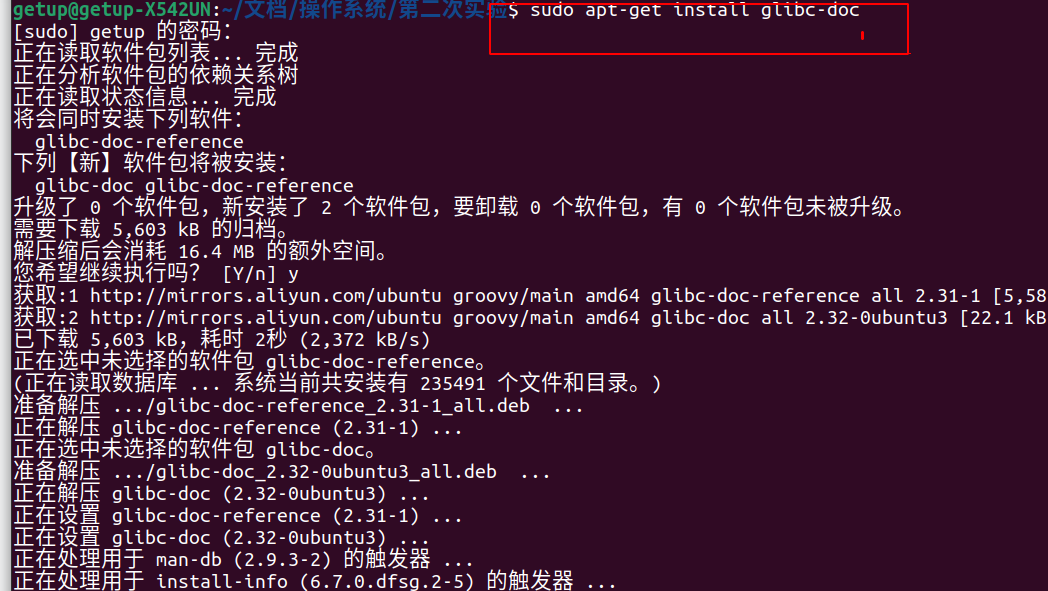sudo apt-get install manpages-posix manpages-posix-dev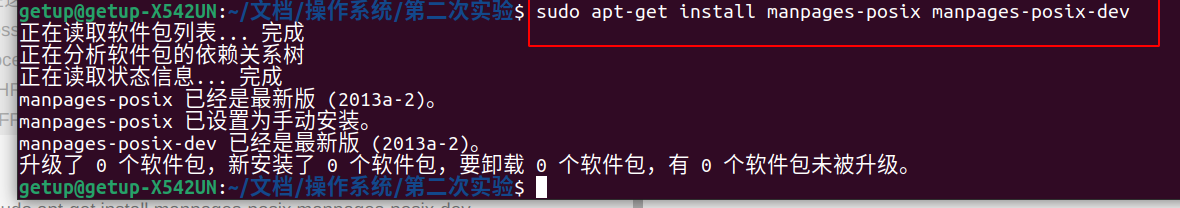vi test2.c

## 4.2代码编写

``````#include<stdio.h>
#include<unistd.h>
#include<stdlib.h>
#include <time.h>

#define random_1(a,b) ((rand()%(b-a))+a)  //随机值将含a不含b
int value = 0;
long double valid=0,all=999999;//初始化有效点为0,总点数为999999
long double pi=0;
void*  fun(void* arg) {
long double x,y;//定义坐标x,y
int i=1;
srand((double)time(NULL)); //设置随机数种子，防止每次产生的随机数相同

for(i=1;i<=999999;i++)
{
//获取x,y的随机值,0<=x<100000,0<=y<100000
x=random_1(0,100000);
y=random_1(0,100000);
if(x*x+y*y<=10000000000)
valid++;
}
return (void*)0;
}
//主函数
int main()
{
clock_t start, end;
start = clock();  //读取程序开始运行的时间
int error;//定义线程返回值error,若是成功建立线程返回0,否则返回错误的编号
sleep(2);//用sleep()控制子线程的不同并发过程

if(error) {
exit(1);
}
if(error) {
exit(1);
}
end = clock();           //记录结束时间
pi=valid/999999*4;
printf("valid的值为： %Lf\n",valid);
printf("总的点数的值为999999\n");
printf("π的值为： %Lf\n",pi);
double seconds  =(double)(end - start)/CLOCKS_PER_SEC*1000;
printf("Use time is: %.3fms\n", seconds);
//进程锁销毁
return 0;
}

``````

## 4.3执行代码

./test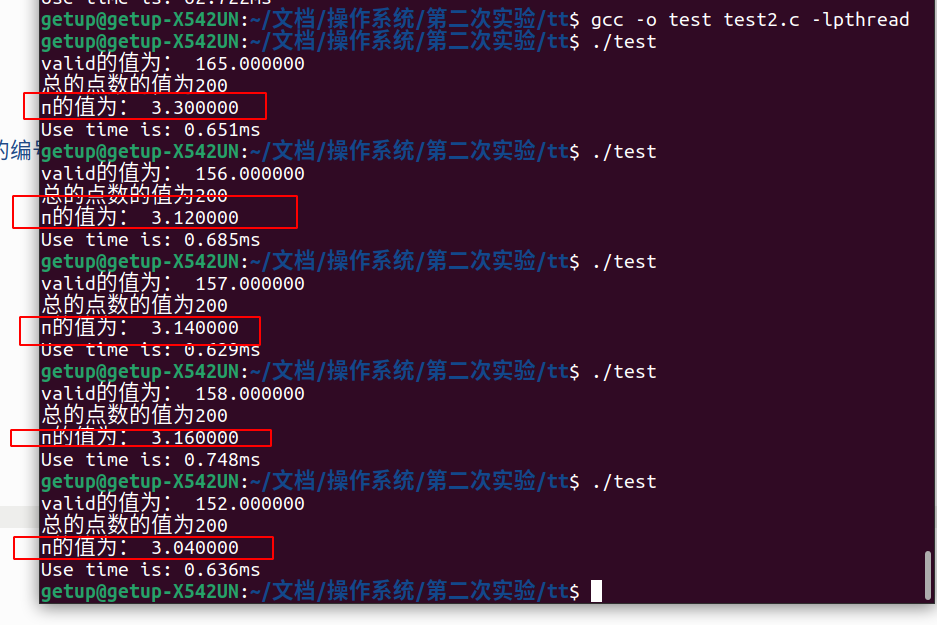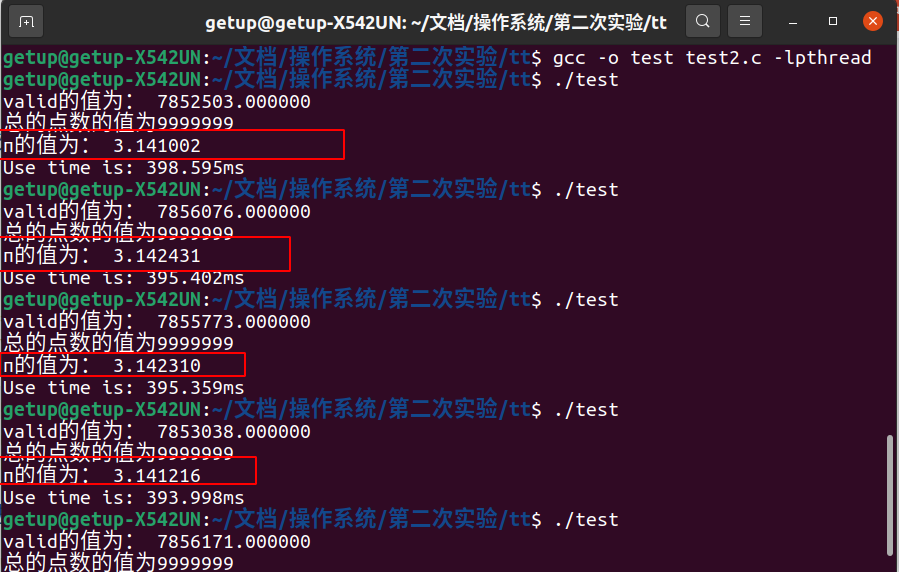//可修改部分参数使结果更精确（多次测量求平均值）

# 6实验改进：OpenMP方法

## 6.1 OpenMP方法介绍

Open Multi-Processing的缩写，是一个应用程序接口（API），可用于显式指导多线程、共享内存的并行性。

OpenMP支持的语言包括C/C++、Fortran；而支持OpenMP的编译器VS、gcc、clang等都行。可移植性也很好：Unix/Linux和Windows

## 6.2 编程实现

vi test2_2.c

``````#include<stdio.h>
#include<unistd.h>
#include<stdlib.h>
#include <time.h>
#include<omp.h>
#define random_1(a,b) ((rand()%(b-a))+a)  //随机值将含a不含b
int value = 0;
long double valid=0,all=999999;//初始化有效点为0,总点数为999999
long double pi=0;
//void*  fun(void* arg) {
void fun(){

long double x,y;//定义坐标x,y

int i=1;

srand((double)time(NULL)); //设置随机数种子，防止每次产生的随机数相同

for(i=1;i<=999999;i++)
{

//获取x,y的随机值,0<=x<100000,0<=y<100000
x=random_1(0,100000);
y=random_1(0,100000);
if(x*x+y*y<=10000000000)
#pragma omp crirical         //OpenMP并行编排支持
{
valid++;
}
}
//  return (void*)0;
}
//主函数
int main()
{
clock_t start, end;
start = clock();  //读取程序开始运行的时间

fun();
end = clock();           //记录结束时间
pi=valid/999999*4;
printf("valid的值为： %Lf\n",valid);
printf("总的点数的值为999999\n");
printf("π的值为： %Lf\n",pi);
double seconds  =(double)(end - start)/CLOCKS_PER_SEC*1000;
printf("Use time is: %.3fms\n", seconds);
//进程锁销毁
return 0;
}

``````

gcc -o test test2_2.c -fopenmp

./test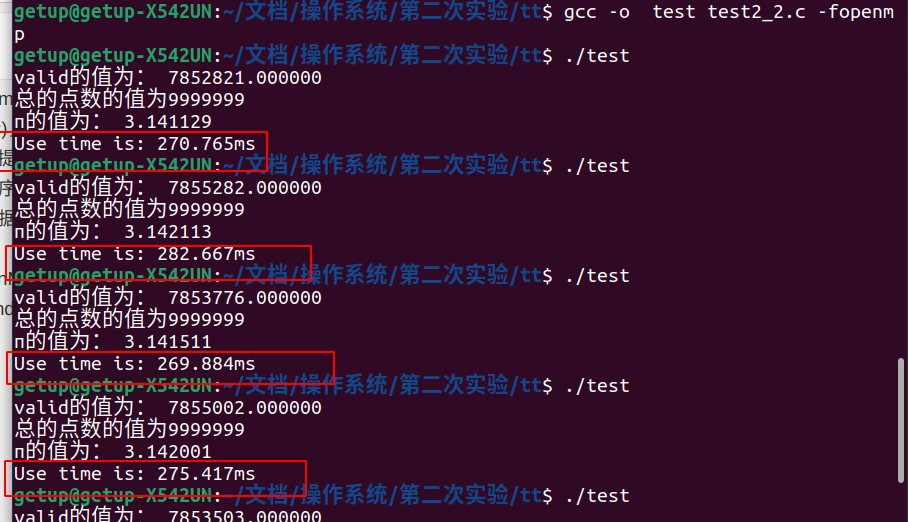# 7源代码下载帖子
视频
声望
粉丝
热门推荐
社区精华内容